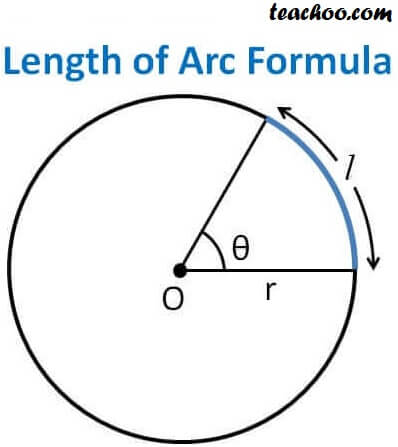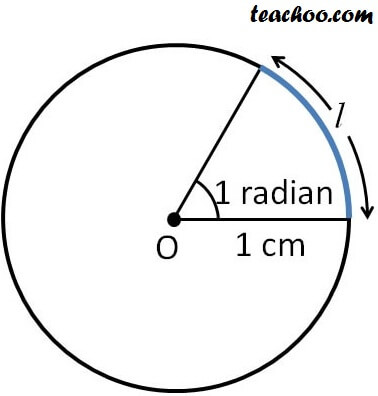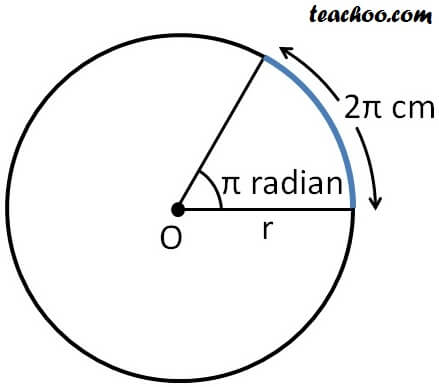Arc length

Chapter 3 Class 11 Trigonometric Functions
Concept wise

Let us consider a circle with radius rArc is a portion of the circle.

Let the arc subtend angle θ at the center

Then,

Angle at center = Length of Arc/ Radius of circle

θ = l/r

Note: Here angle is in radians.

Let’s take some examples

### If radius of circle is 5 cm, and length of arc is 12 cm. Find angle subtended by arc-a-

Given radius = r = 5 cm

Length of arc = l = 12 cm

We know that

θ = l /r

θ = 12/5

∴ Angle subtended by angle arc = 12/5 radians

-ea-

### If angle subtended by arc is 1 radian, and radius of circle is 1 cm. Find length of arc-a-

Given radius = r = 1 cm

Angle = θ = 1 radian

We know that

θ = l /r

1 = l /1

1 = l

l = 1 cm

∴ Length of the arc = 1 cm

-ea-

### If angle subtended by arc is π radian, and length of the arc is 2 π cm. What is the radius of circle?-a-

Given

Length of arc = l = 2π cm

Angle = θ = π radian

We know that

θ = l /r

π = 2π/r

r = 2π/π

r = 2 cm

∴ Radius of circle = 2 cm

-ea-

Learn in your speed, with individual attention - Teachoo Maths 1-on-1 Class

### Transcript